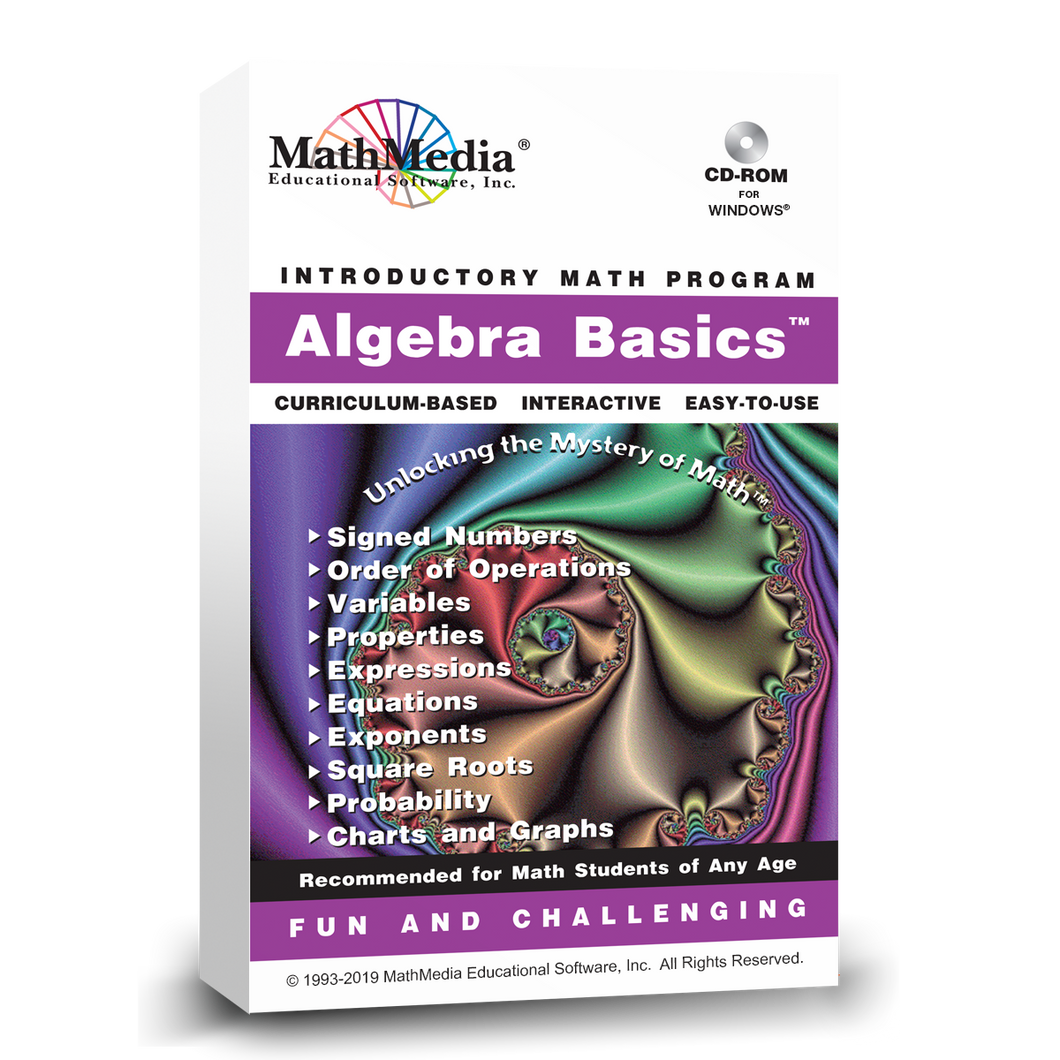# Algebra Basics™

Regular price \$49.00 \$12.25 Sale

Pre-Algebra is the single most important pre-requisite for success in higher math courses (Algebra 1 & 2, Geometry, Trigonometry, Pre­Calculus, and Calculus). The distance covered on the math course continuum is dependent upon the student’s preparation for their first theoretical math class, first year Algebra.

Pre-Algebra is a basic necessity to being properly prepared for high school algebra. The more foundation a math student has, the better chance he/she will progress successfully through their high school years of math education. Of all the math concepts necessary for further and more advanced study of math, the foundation of understanding pre-algebra is crucial. Learning Basic Algebra with this software works. This pre algebra program offers basic algebra help with instruction and practice basic algebra problems on the topics necessary for success in high school Algebra. Immediate feedback with automatic scoring for students and teachers is available to track progress.

Print Product DescriptionThis is the 4th program in the Basic Math Series.
This basic algebra material is usually taught to middle school math students but is also very appropriate for adult learners returning to their math education. This basic algebra software is written in a very straight forward manner which appeals to middle school math students, high school students, and adults.

MathMedia pre-algebra tutorials include: Signed Numbers, Order of Operations, Variables, Properties, Expressions, Equations, Exponents, Square Roots, Sets, Probability, and Charts and Graphs. Pre-Algebra is the foundation for success in future math classes. MathMedia provides basic algebra lessons and practice for the beginning algebra student. Short quizzes are available for assessing each topic.

TEST: This basic algebra learning system provides two tests which are scored and stored by the computer. Test A may be used as a pre-test for placement purposes and Test B may be used for accountability after all the lessons and quizzes have been completed. A printout of the student's answers and and evaluation of which sections require additional learning is provided. The student then returns to the body of the program to receive the algebra help they need to have basic algebra skills.

This pre-algebra program is for the beginning algebra student - this is one program from the MathMedia middle school "Basic Math Series" which includes five programs: Fraction Basics, Decimal Basics, Percent Basics, Algebra Basics, and Geometry Basics.

Use this tutorial software to keep up with schoolwork, to learn at home, to remediate, to accelerate, as a course or as a reference.